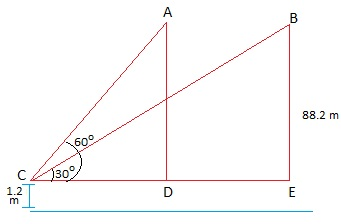Guru

# A 1.2 m tall girl spots a balloon moving with the wind in a horizontal line at a height of 88.2 m from the ground. The angle of elevation of the balloon from the eyes of the girl at any instant is 60°. After some time, the angle of elevation reduces to 30° (see Fig. 9.13). Find the distance travelled by the balloon during the interval. Q.14

• 0

How i solve this problem in easy way for the question from class 10th of chapter Some applications of Trigonometry of Exercise 9.1 of question no.14, give me the best way to solve this problem A 1.2 m tall girl spots a balloon moving with the wind in a horizontal line at a height of 88.2 m from the ground. The angle of elevation of the balloon from the eyes of the girl at any instant is 60°. After some time, the angle of elevation reduces to 30° (see Fig. 9.13). Find the distance travelled by the balloon during the interval.

Share

1. Let the initial position of the balloon be A and final position be B.

Height of balloon above the girl height = 88.2 m – 1.2 m = 87 m.

To Find: Distance travelled by the balloon = DE = CE – CD

Let us redesign the given figure as per our convenientStep 1: In right ΔBEC,

tan 30° = BE/CE

1/√3= 87/CE

CE = 87√3

Step 2: